Private GMAT Tutoring in NYC & Online

GMAT Question of the Day - Problem Solving - Combinatorics/Number Properties

A certain company tags each of its products with an 8 digit alpha numeric code whose first 4 digits are either a digit 0-9 or a letter of the alphabet and whose last 4 digits represent the year of manufacture (for example, the last 4 digits of a product created in 2010 would be 2010). If letters and numbers may be repeated what is the maximum number of products that can be given a unique tag in any 3 year period?

A. 5,038,848

B. 3,020,176

C. 1,679,616

D. 1,513,692

E. 1,217,460

GMAT Question of the Day Solution

Here is another challenging GMAT combinatorics question. If you have a good basic understanding of ordering this question of the day shouldn't be too difficult. The most difficult part is understanding the parameters of the question. What are the rules for the code? Once you've done that this is a basic ordering question (for my GMAT tutoring students I recommend a simple slot method which you can see in the explanation diagram) with just one more twist: The numbers are huge! Whenever you have a seemingly impossible calculation the GMAT will help you out with a shortcut. One to look out for - units digit. You can do some quick and dirty units digit math and see that only one answer choice has the appropriate units digit.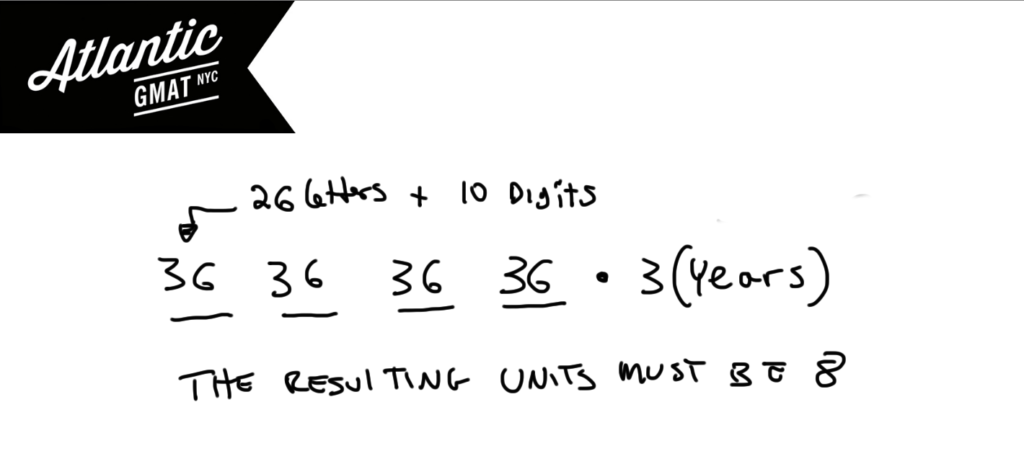GMAT Question of the Day - Problem Solving - Combinatorics

How many unique ways can 7 people be arranged around a circular table (The same relative arrangement does not count as unique)?

A. 7!

B. 6!

C. 5!

D. 7!/5!

E. 7!/4!

GMAT Question of the Day Solution

Most GMAT combinations/permutations questions are not difficult but many GMAT tutoring students are worried about these topics. Most likely you won't see many of these on your GMAT. But still it's worth knowing the basics. This GMAT question of the day focuses on a specific type of permutation: the circular arrangement.

Circular arrangements are different than straight line arrangements in that the question will generally specify that if the same relative positions are maintained around the circle then the arrangement has not changed. Meaning if you're sitting in between Bill and Bob and then everyone moves one chair to the left and you are still between Bill and Bob that's not a new arrangement. How do you take this into account? Simple: subtract one from the total and then take the factorial. In this case (7-1)! or 6!.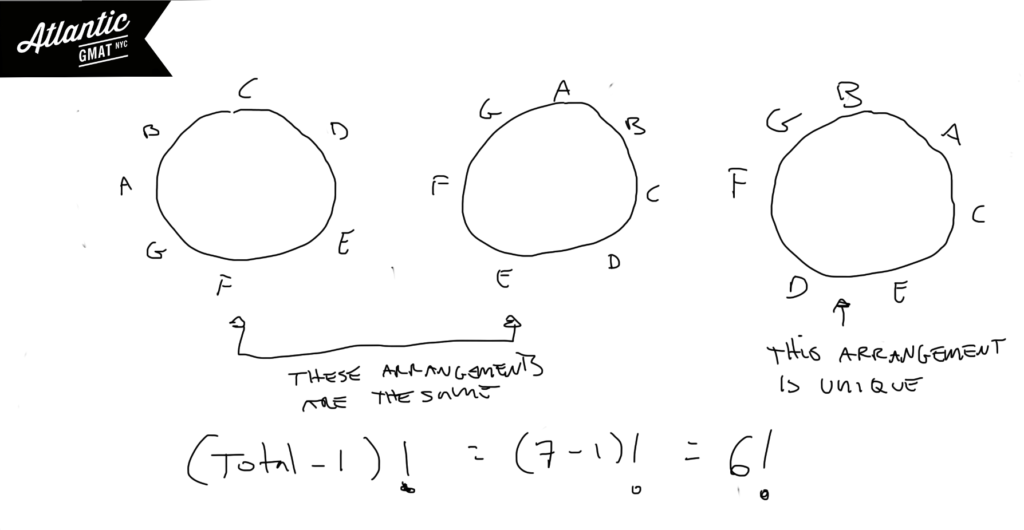GMAT Question of the Day - Problem Solving - Probability

Katie has a bag of marbles which contains 3 blue marbles, 3 red marbles, and 2 yellow marbles. If she arranges her marbles in a line what is the probability that the marbles will be grouped by color?

A. 3/280

B. 3/560

C. 5/632

D. 7/632

E. 7/635

GMAT Question of the Day Solution

GMAT probability can feel very easy or near impossible depending on your mastery of a few simple rules. Probability breaks down to:

Specific Scenario (in this case the marbles arranged by color)/Total Scenarios (in this case the total ways the marbles can arranged). It's important to realize that when calculating the total ways that the marbles can be arranged that switching two red marbles does not make a new arrangement (or switching two greens or switching two yellows). We need to use the non-unique ordering formula. The factorial of the total items (in this case 8 marbles so 8!) divided by the factorial of each individual item (3!, 3!, 2!). Any time that you are arranging/ordering non-unique items you need to use this formula.

Also realize that with the specific scenario the real question is: how many ways can you arrange three different colored marbles? Why? Well, you have to group each color so really each group just represents a color. Arranging three unique things is just 3!

Quick note on arithmetic. Notice in the solution that I didn't multiply 8, 7, 5, and 2 but waited until the very end so that I could simplify more. On the GMAT it's helpful to wait on multiplication until you are sure that you won't be able to simplify further. The GMAT usually gives you a way to keep your arithmetic manageable. Get in the habit of picking up on these cues.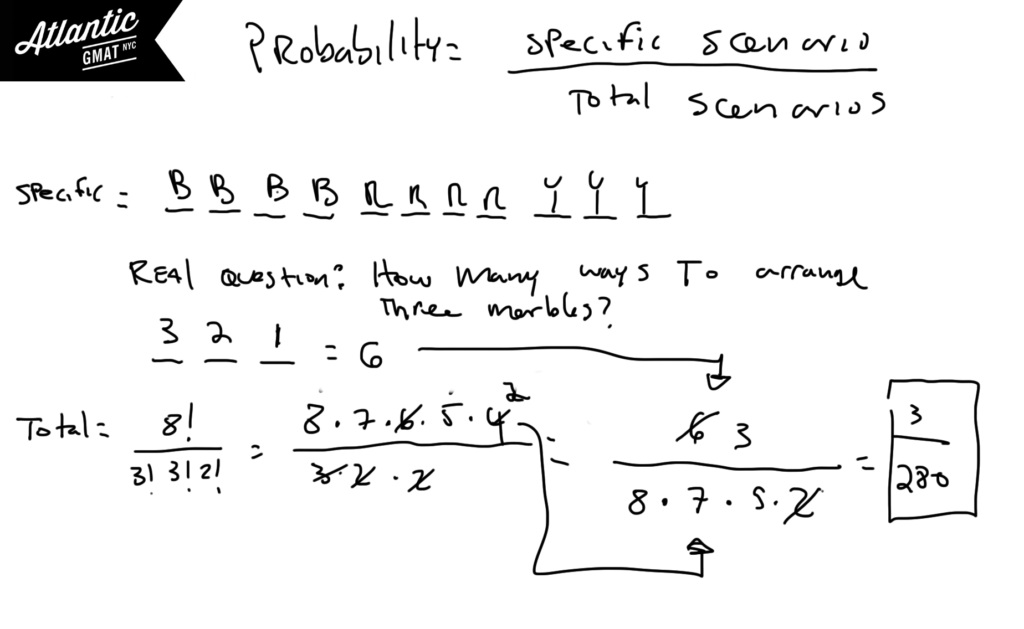GMAT Question of the Day - Problem Solving - Combinations/Permutations

An animal shelter is producing unique tags for each of the x number of animals at the shelter. Each tag will have a code made up of two letters and two digits with no letters or digits repeating. If there are exactly the same number of animals as there are codes then what is the value of x?

A. 46430

B. 48690

C. 52864

D. 56862

E. 58500

GMAT Question of the Day Solution:

In this question we're being asked to make codes. That means the order matters. This is the same as figuring out how many different ways you can line up a group of children, how many ways a group of people can walk through a door, or how many combinations you can make for a lock given a certain number of numbers. The slot method works well for this. Also, you've got some pretty big numbers and therefore some potentially long calculations. Look for ways to simplify your work. There is a 10 in the multiplication so the answer must end in 0. You can also do some rough arithmetic using easy calculations (25*25*10*9) and set a lower limit for the answer. See the diagram below: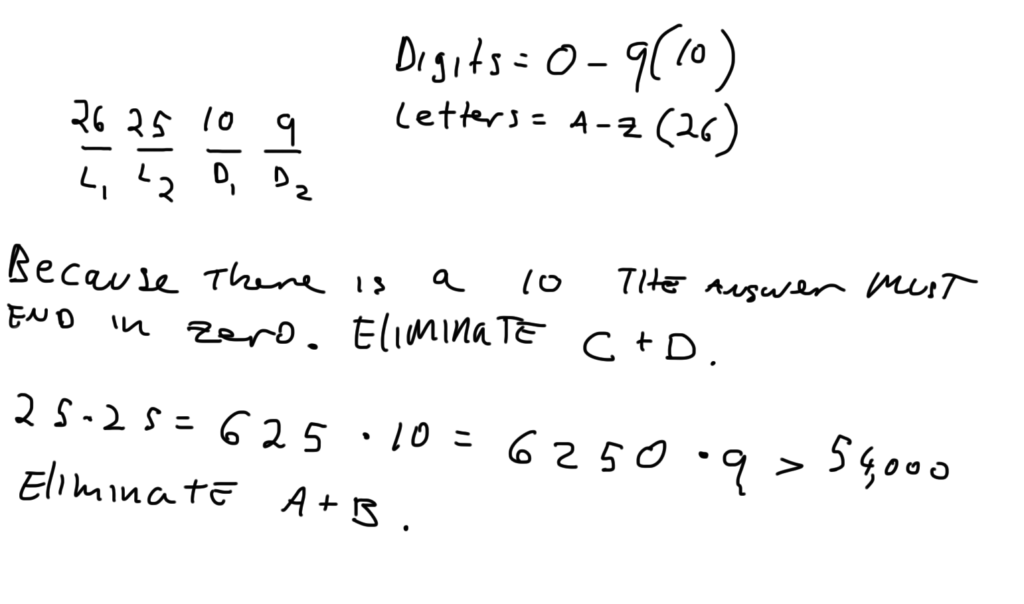Atlantic GMAT Question of the Day - PS Combinations - April 28th, 2014

The buyer of a new car can choose from 5 different colors, 3 engine types, and either a basic or deluxe sound system. If Bill is planning to buy two new cars how many different combinations of the two cars are possible so that no pair of cars has the same engine?

A. 200

B. 300

C. 400

D. 500

E. 600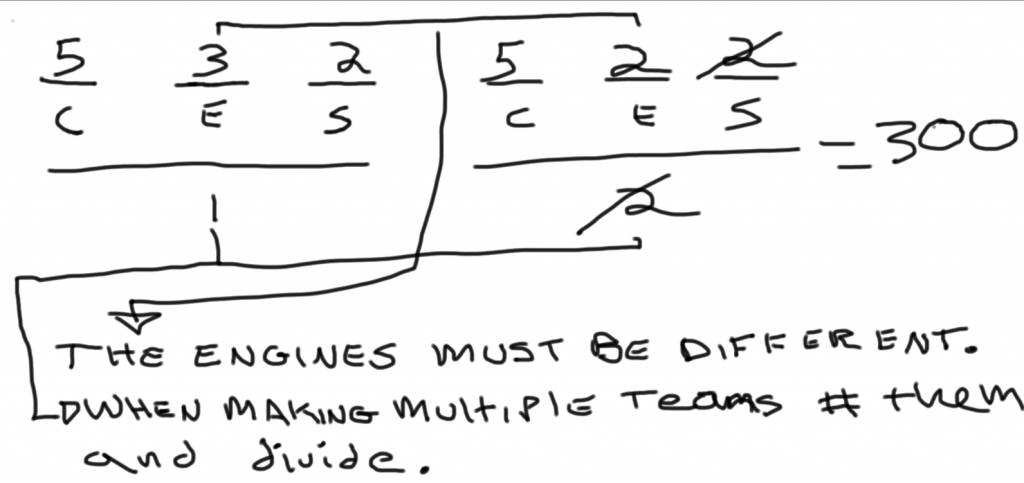CONTACT

Atlantic GMAT

405 East 51st St.

NY, NY 10022

(347) 669-3545

info@AtlanticGMAT.com